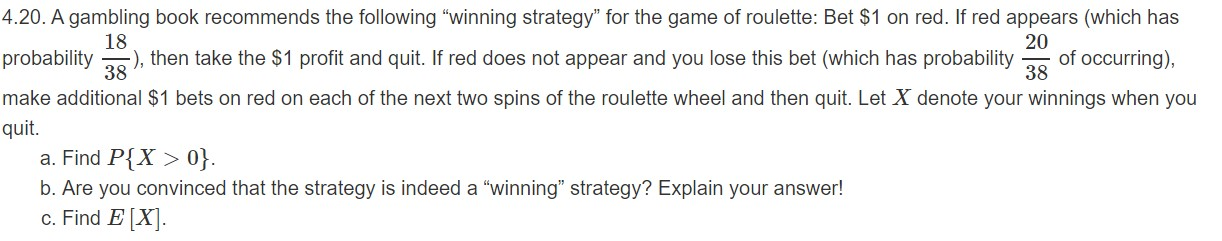# 4.20. A gambling book recommends the following "winning strategy" for the game of roulette: Bet \$1 on red. If red appears (which has18), then take the \$1 profit and quit. If red does not appear and you lose this bet (which has probability20of occurring),38probability38make additional \$1 bets on red on each of the next two spins of the roulette wheel and then quit. Let X denote your winnings when youquit.a. Find P{X> 0}.b. Are you convinced that the strategy is indeed a "winning" strategy? Explain your answer!c. Find E[X

Question
69 viewshelp_outlineImage Transcriptionclose4.20. A gambling book recommends the following "winning strategy" for the game of roulette: Bet \$1 on red. If red appears (which has 18 ), then take the \$1 profit and quit. If red does not appear and you lose this bet (which has probability 20 of occurring), 38 probability 38 make additional \$1 bets on red on each of the next two spins of the roulette wheel and then quit. Let X denote your winnings when you quit. a. Find P{X> 0}. b. Are you convinced that the strategy is indeed a "winning" strategy? Explain your answer! c. Find E[X fullscreen
check_circle

star
star
star
star
star
1 Rating
Step 1

X- be the winnings when we quit.
Case-1 win in first spin
Probability of win in first spin = 18/38
profit when you quit X = \$1

Case-2 loss in first spin
probability of loss in first spin = 20/38

Possible outcomes in next two spins are (win, win), (win loss), (loss,win),(loss,loss)
probability of win in third spin  and win in fourth spin= (18/38)(18/38)
profit when you quit X = -1+1+1 = 1

probability of win in third spin  and loss in fourth spin= (18/38)(20/38)
profit when you quit = -1+1-1 = -1

probability of loss in third spin  and win in fourth spin= (20/38)(18/38)
profit when you quit = -1-1+1 = -1

probability of loss in third spin  and loss in fourth spin= (20/38)(20/38)
profit when you quit = -1-1-1 = -3

Possible outocmes of X are 1,1,-1,-1,-3 with probabilities as shown below

Step 2

P(X>0) is calculated as shown below,
we get P(X > 0) = 0.592 (rounded to three decimals).

Step 3

The probability of net profit greater than 0 is 0.592. If we consider this probability we can say that the chance to quit the roulette table with winning will be favourable as it is more than...

### Want to see the full answer?

See Solution

#### Want to see this answer and more?

Solutions are written by subject experts who are available 24/7. Questions are typically answered within 1 hour.*

See Solution
*Response times may vary by subject and question.
Tagged in

### Measures